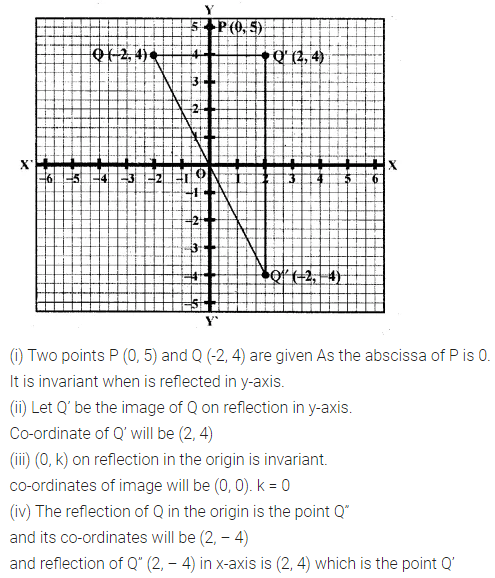# ML Aggarwal Class 10 Solutions for ICSE Maths Chapter 10 Reflection Ex 10

## ML Aggarwal Class 10 Solutions for ICSE Maths Chapter 10 Reflection Ex 10

ML Aggarwal Class 10 Solutions for ICSE Maths Chapter 10 Reflection Ex 10

Question 1.
Find the co-ordinates of the images of the following points under reflection in the x- axis:
(i) (2, -5)
(ii) $$-\frac { 3 }{ 2 } ,-\frac { 1 }{ 2 }$$
(iii) (-7, 0)
Solution:Question 2.
Find the co-ordinates of the images of the following points under reflection in the y-axis:
(i) (2, -5)
(ii) $$-\frac { 3 }{ 2 } ,\frac { 1 }{ 2 }$$
(iii) (0, -7)
Solution: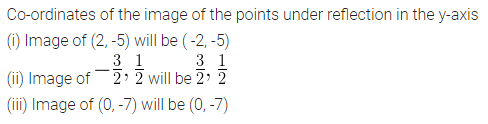Question 3.
Find the co-ordinates of the images of the following points under reflection in the origin:
(i) (2, -5)
(ii) $$\frac { -3 }{ 2 } ,\frac { -1 }{ 2 }$$
(iii) (0, 0)
Solution: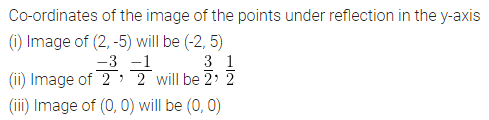Question 4.
The image of a point P under reflection in the x-axis is (5, -2). Write down the coordinates of P.
Solution:Question 5.
A point P is reflected in the x-axis. Co-ordinates of its image are (8, -6).
(i) Find the co-ordinates of P.
(ii) Find the co-ordinates of the image of P under reflection in the y-axis.
Solution:Question 6.
A point P is reflected in the origin. Co-ordinates of its image are (2, -5). Find
(i) the co-ordinates of P.
(ii) the co-ordinates of the image of P in the x-axis.
Solution:Question 7.
(i) The point P (2, 3) is reflected in the line x = 4 to the point P’. Find the co-ordinates of the point P’.
(ii) Find the image of the point P (1, -2) in the line x = -1.
Solution: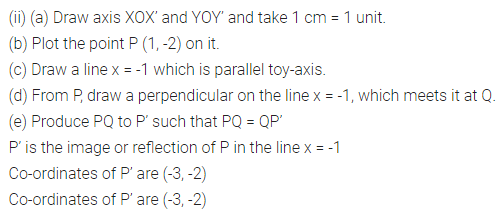Question 8.
(i) The point P (2, 4) on reflection in the line y = 1 is mapped onto P’ Find the co-ordinates of P’.
(ii) Find the image of the point P ( -3, -5) in the line y = -2.
Solution:Question 9.
The point P ( -4, -5) on reflection in y-axis is mapped on P’. The point P’ on reflection in the origin is mapped on P”. Find the co-ordinates of P’ and P”. Write down a single transformation that maps P onto P”.
Solution:Question 10.
Write down the co-ordinates of the image of the point (3, -2) when:
(i) reflected in the x-axis
(ii) reflected in the y-axis
(iii) reflected in the x-axis followed by a reflection in the y-axis
(iv) reflected in the origin. (2000)
Solution: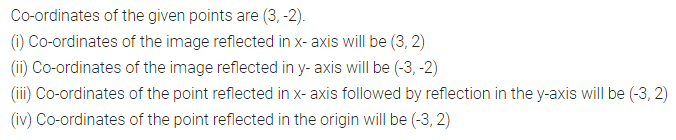Question 11.
Find the co-ordinates of the image of (3, 1) under reflection in x-axis followed by a reflection in the line x = 1.
Solution:Question 12.
If P’ (-4, -3) is the image of a point P under reflection in the origin, find
(i) the co-ordinates of P.
(ii) the co-ordinates of the image of P under reflection in the line y = -2.
Solution:Question 13.
A Point P (a, b) is reflected in the x-axis to P’ (2, -3), write down the values of a and b. P” is the image of P, when reflected in the y-axis. Write down the co-ordinates of P”. Find the co-ordinates of P”, when P is reflected in the line parallel to y-axis such that x = 4. (1998)
Solution: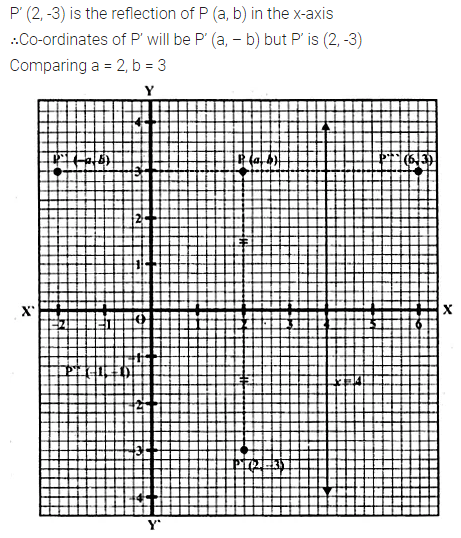Question 14.
(i) Point P (a, b) is reflected in the x-axis to P’ (5, -2). Write down the values of a and b.
(ii) P” is the image of P when reflected in the y-axis. Write down the co-ordinates of P”.
(iii) Name a single transformation that maps P’ to P”. (1997)
Solution:Question 15.
Points A and B have co-ordinates (2, 5) and (0, 3). Find
(i) the image A’ of A under reflection in the x-axis.
(ii) the image B’ of B under reflection in the line AA’.
Solution:Question 16.
Plot the points A (2, -3), B (-1, 2) and C (0, -2) on the graph paper. Draw the triangle formed by reflecting these points in the x-axis. Are the two triangles congruent?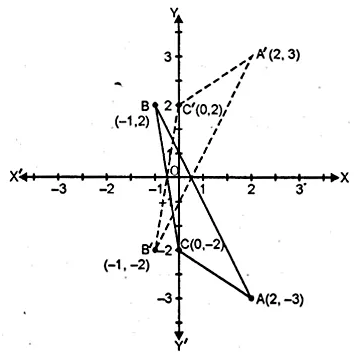Solution:Question 17.
The points (6, 2), (3, -1) and (-2, 4) are the vertices of a right-angled triangle. Check whether it remains a right-angled triangle after reflection in the y-axis.
Solution: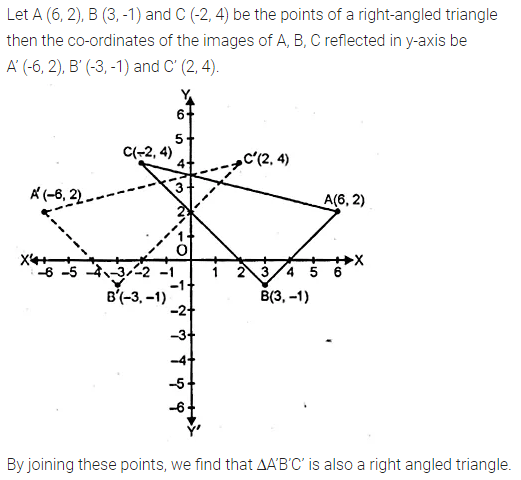Question 18.
The triangle ABC where A (1, 2), B (4, 8), C (6, 8) is reflected in the x-axis to triangle A’ B’ C’. The triangle A’ B’ C’ is then reflected in.the origin to triangle A”B”C” Write down the co-ordinates of A”, B”, C”. Write down a single transformation that maps ABC onto A” B” C”.
Solution: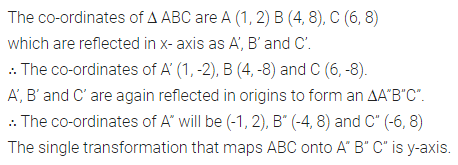Question 19.
The image of a point P on reflection in a line l is point P’. Describe the location of the line l.
Solution: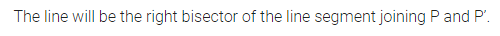Question 20.
Given two points P and Q, and that (1) the image of P on reflection in the y-axis is the point Q and (2) the midpoint of PQ is invariant on reflection in x-axis. Locate
(i) the x-axis
(ii) the y-axis and
(iii) the origin.
Solution:Question 21.
The point (-3, 0) on reflection in a line is mapped as (3, 0) and the point (2, -3) on reflection in the same line is mapped as (-2, -3).
(i) Name the mirror line.
(ii) Write the co-ordinates of the image of (-3, -4) in the mirror line.
Solution:Question 22.
A (-2, 4) and B (-4, 2) are reflected in the y-axis. If A’ and B’ are images of A and B respectively, find(i) the co-ordinates of A’ and B’.
(ii) Assign a special name to a quad. AA’B’B.
(iii) State whether AB’ = BA’.
Solution:Question 23.
Use graph paper for this question.
(i) The point P (2, -4) is reflected about the line x = 0 to get the image Q. Find the co-ordinates of Q.
(ii) Point Q is reflected about the line y = 0 to get the image R. Find the co-ordinates of R.
(iii) Name the figure PQR.
(iv) Find the area of figure PQR. (2007)
Solution:Question 24.
Use graph paper for this question. The point P (5, 3) was reflected in the origin to get the image P’.
(i) Write down the co-ordinates of P’.
(ii) If M is the foot of the perpendicular from P to the x-axis, find the co-ordinates of M.
(iii) If N is the foot of the perpendicular from P’ to the x-axis, find the co-ordinates of N.
(iv) Name the figure PMP’N.
(v) Find the area of the figure PMP’N. (2001)
Solution:Question 25.
Using a graph paper, plot the points A (6, 4) and B (0, 4).
(i) Reflect A and B in the origin to get the images A’ and B’.
(ii) Write the co-ordinates of A’ and B’.
(iii) State the geometrical name for the figure ABA’B’.
(iv) Find its perimeter.
Solution:Question 26.
Use graph paper to answer this question
(i) Plot the points A (4, 6) and B (1, 2).
(ii) If A’ is the image of A when reflected in x-axis, write the co-ordinates of A’.
(iii) If B’ is the image of B when B is reflected in the line AA’, write the co-ordinates of B’.
(iv) Give the geometrical name for the figure ABA’B’. (2009)
Solution:Question 27.
The points A (2, 3), B (4, 5) and C (7, 2) are the vertices of ∆ABC. (2006)
(i) Write down the co-ordinates of A1, B1, C1 if ∆ A1B1C1 is the image of ∆ ABC when reflected in the origin.
(ii) Write down the co-ordinates of A2, B2, C2 if ∆ A2B2C2 is the image of ∆ ABC when reflected in the x-axis.
(iii) Assign the special name to the quadrilateral BCC2B2 and find its area.Solution: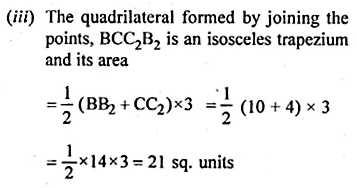Question 28.
The point P (3, 4) is reflected to P’ in the x-axis and O’ is the image of O (origin) in the line PP’. Find:
(i) the co-ordinates of P’ and O’,
(ii) the length of segments PP’ and OO’.
(iii) the perimeter of the quadrilateral POP’O’.
Solution: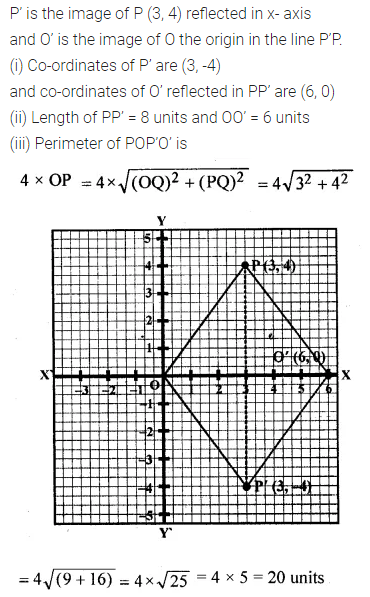Question 29.
Use a graph paper for this question. (Take 10 small divisions = 1 unit on both axes). P and Q have co-ordinates (0, 5) and (-2, 4).
(i) P is invariant when reflected in an axis. Name the axis.
(ii) Find the image of Q on reflection in the axis found in (i).
(iii) (0, k) on reflection in the origin is invariant. Write the value of k.
(iv) Write the co-ordinates of the image of Q, obtained by reflecting it in the origin followed by a reflection in x-axis. (2005)
Solution: